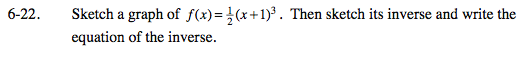### Home > INT3 > Chapter Ch6 > Lesson 6.1.2 > Problem6-22

6-22.

Sketch a graph of f(x) =(x + 1)3. Then sketch its inverse and write the equation of the inverse. Homework Help ✎This function adds 1, cubes, then multiplies by 1/2. How can you undo those operations?

Use a graphing calculator to graph both the function and its inverse. Are they reflections across the line y = x?